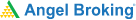# Calculating Volatility: A Simplified Approach## Calculation of Historical Volatility

Since calculation of historical volatility is based on past and concrete data, institutional investors follow a thumb rule when calculating it. It is measured using a variance.

Let’s take a look at an example and see how this is done.

In the table below, we have a dataset containing the closing prices of ABS stocks over a period of 5 weeks:

 Week 1 23 Week 2 21 Week 3 22 Week 4 20 Week 5 24

Step 1: Calculating mean

Mean = (23 + 21 + 22 + 20 + 24)/5 = 110/5 = 22

Step 2: Calculating difference between the mean value and each component in the dataset

Subsequently, it is required to calculate the deviation between 22 and the rest of the elements in that dataset.

23 – 22 = 1

21 – 22 = – 1

22 – 22 = 0

20 – 22 = – 2

24 – 22 = 2

Step 3: Squaring the deviations and adding them

[1^2 + (-1)^2 + 0 + (-2)^2 + 2^2] = 10

Step 4: Dividing the squared deviations by the total number of elements in the dataset to calculate variance (ơ2)

\sigma ^2 = 10/5 = 2

Since it is the variance, the standard variation of the dataset will be found by square rooting this value.

Standard deviation of ABS stocks = √2 = 1.414

However, this process only holds in case of uniform distribution. In the case of random sampling from a voluminous dataset, only 68% of this data agrees with or falls within the standard deviation mentioned above.

## Calculating Volatility

Volatility is the statistical measure of the dispersion of returns during a certain period of time for stocks, security, or market index. It can be calculated using standard deviation or the variance of the security or stock.

The easiest way to calculate the volatility of a security is to calculate the standard deviation of its prices over a period of time. This can be done by using the following steps:

• ### Daily Volatility Formula:

The formula for daily volatility is computed by finding out the square root of the variance of a daily stock price.

Daily Volatility formula = √Variance

Next, the annualized volatility formula is calculated by multiplying the daily volatility by a square root of 252.

Learning & Earning is now super simple₹ 0 Equity Delivery

No Hidden Charges₹ 20 Per Order For Intraday

FAQ,Currencies & CommoditiesZERO Brokerage*

on ALL SegmentsFREE Margin

Trade Funding

• ### Annualized Volatility Formula:

Annualized Volatility Formula = √252 * √Variance

The formula for the volatility of a particular stock can be derived by using the following steps:

Step 1: Gather daily stock price and determine the mean of the stock price. Let us assume the daily stock price on an ith day as Pi and the mean price as Pav.

Step 2: Calculate the difference between each day’s stock price and the mean price, i.e., Pi – P.

Step 3: Calculate the square of all the deviations, i.e. (Pav – Pi)2.

Step 4: Find the summation of all the squared deviations, i.e. ∑ (Pav – Pi)2.

Step 5: Divide the summation of all the squared deviations by the number of daily stock prices, say n. It is called the variance of the stock price.

Variance = ∑ (Pav – Pi)2 / n

Step 6: Calculate the daily volatility or standard deviation by calculating the square root of the variance of the stock.

Daily volatility = √(∑ (Pav – Pi)2 / n)

Step 7: The annualized volatility formula is calculated by multiplying the daily volatility by the square root of 252. Here, 252 is the number of trading days in a year.

Annualized volatility = = √252 * √(∑ (Pav – Pi)2 / n)

#### Wrapping up

Relevance of the Formulas:

Understanding the concept of volatility is integral as it refers to the measure of risk relating to the quantum of changes in the value of a security or stock. Higher volatility means that the stock value of the stock can be spread out over a larger range of values. This means that the value of the stock can potentially move in either direction over a short period. On the other hand, lower volatility indicates that the value of the stock would not fluctuate much and will continue to remain stable over the period.

#### A Quick Recap

1. Historical volatility is measured using a variance.
2. Using mean to calculate historical volatility only holds in the case of uniform distribution.
3. Volatility is the statistical measure of the dispersion of returns during a certain period of time for stocks, security, or market index.
4. The easiest way to calculate the volatility of a security is to calculate the standard deviation of its prices over a period of time.Test Your Knowledge

Take the quiz for this chapter & mark it complete.

Comments (0)

Add Comment

Get Information Mindfulness!

Catch-up With Market

News in 60 Seconds.The perfect starter to begin and stay tuned with your learning journey anytime and anywhere.Ready To Trade? Start withOpen an account